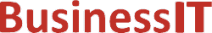O nás     Inzerce     KontaktSpolehlivé informace o IT již od roku 2011[Linux manuál]

# tan, tanf, tanl: tečná funkce

Originální popis anglicky: tan, tanf, tanl - tangent function

Návod, kniha: POSIX Programmer's Manual

## STRUČNĚ

#include <math.h>

double tan(double x);

float tanf(float x);

long double tanl(long double x);

## POPIS / INSTRUKCE

These functions shall compute the tangent of their argument x, measured in radians.
An application wishing to check for error situations should set errno to zero and call feclearexcept(FE_ALL_EXCEPT) before calling these functions. On return, if errno is non-zero or fetestexcept(FE_INVALID | FE_DIVBYZERO | FE_OVERFLOW | FE_UNDERFLOW) is non-zero, an error has occurred.

## NÁVRATOVÁ HODNOTA

Upon successful completion, these functions shall return the tangent of x.
If the correct value would cause underflow, and is not representable, a range error may occur, and  either 0.0 (if supported), or  an implementation-defined value shall be returned.
If x is NaN, a NaN shall be returned.
If x is ±0, x shall be returned.
If x is subnormal, a range error may occur and x should be returned.
If x is ±Inf, a domain error shall occur, and either a NaN (if supported), or an implementation-defined value shall be returned.
If the correct value would cause underflow, and is representable, a range error may occur and the correct value shall be returned.
If the correct value would cause overflow, a range error shall occur and tan(), tanf(), and tanl() shall return ±HUGE_VAL, ±HUGE_VALF, and ±HUGE_VALL, respectively, with the same sign as the correct value of the function.

## CHYBY / ERRORY

These functions shall fail if:
Domain Error
The value of x is ±Inf.
If the integer expression (math_errhandling & MATH_ERRNO) is non-zero, then errno shall be set to [EDOM]. If the integer expression (math_errhandling & MATH_ERREXCEPT) is non-zero, then the invalid floating-point exception shall be raised.
Range Error
The result overflows
If the integer expression (math_errhandling & MATH_ERRNO) is non-zero, then errno shall be set to [ERANGE]. If the integer expression (math_errhandling & MATH_ERREXCEPT) is non-zero, then the overflow floating-point exception shall be raised.

These functions may fail if:
Range Error
The result underflows,  or the value of x is subnormal.
If the integer expression (math_errhandling & MATH_ERRNO) is non-zero, then errno shall be set to [ERANGE]. If the integer expression (math_errhandling & MATH_ERREXCEPT) is non-zero, then the underflow floating-point exception shall be raised.

The following sections are informative.

## Taking the Tangent of a 45-Degree Angle

```
#include <math.h>
...
double radians = 45.0 * M_PI / 180;
double result;
...

```

## APPLICATION USAGE

There are no known floating-point representations such that for a normal argument, tan( x) is either overflow or underflow.
These functions may lose accuracy when their argument is near a multiple of pi/2 or is far from 0.0.
On error, the expressions (math_errhandling & MATH_ERRNO) and (math_errhandling & MATH_ERREXCEPT) are independent of each other, but at least one of them must be non-zero.

None.

None.

## SOUVISEJÍCÍ

atan() , feclearexcept() , fetestexcept() , isnan() , the Base Definitions volume of IEEE Std 1003.1-2001, Section 4.18, Treatment of Error Conditions for Mathematical Functions, <math.h> Portions of this text are reprinted and reproduced in electronic form from IEEE Std 1003.1, 2003 Edition, Standard for Information Technology -- Portable Operating System Interface (POSIX), The Open Group Base Specifications Issue 6, Copyright (C) 2001-2003 by the Institute of Electrical and Electronics Engineers, Inc and The Open Group. In the event of any discrepancy between this version and the original IEEE and The Open Group Standard, the original IEEE and The Open Group Standard is the referee document. The original Standard can be obtained online at http://www.opengroup.org/unix/online.html .
 2003 IEEE/The Open Group
©2011-2020 BusinessIT.cz, ISSN 1805-0522 | Názvy použité v textech mohou být ochrannými známkami příslušných vlastníků.
Provozovatel: Bispiral, s.r.o., kontakt: BusinessIT(at)Bispiral.com | Inzerce: Best Online Media, s.r.o., zuzana@online-media.cz
O vydavateli | Pravidla webu BusinessIT.cz a ochrana soukromí | pg(8416)